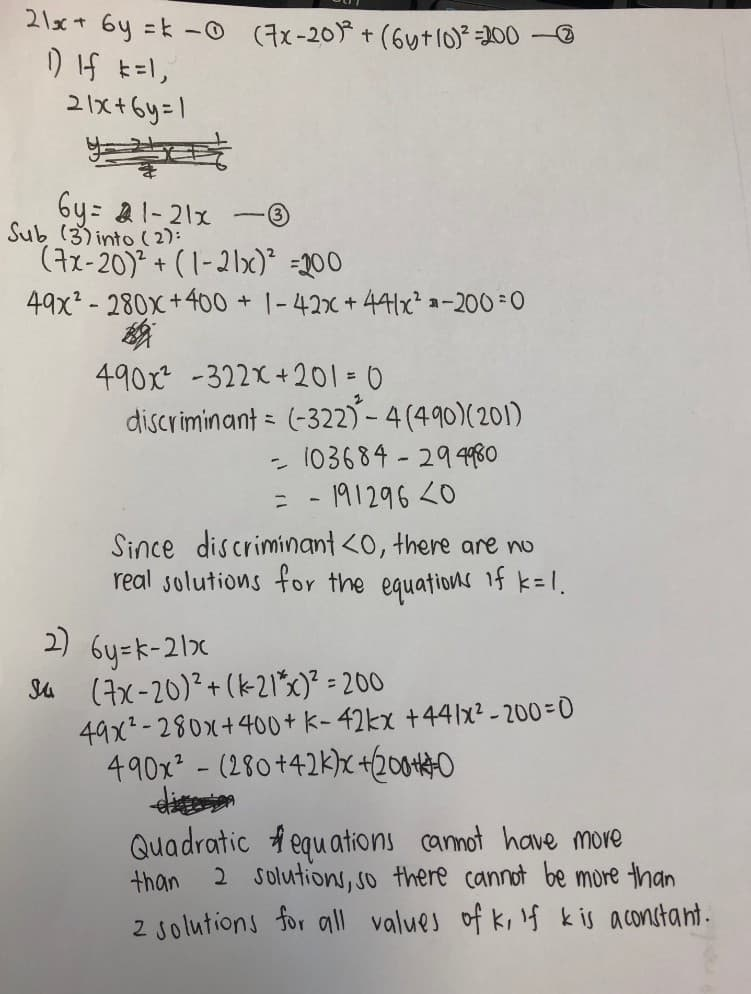# Question

Hi, need help on the following AMaths question:

It is given that 21x + 6y = k and (7x-20)2 + (6y+10)2 = 200. If k = 1,

1) Explain how you could tell that there are no solutions without actually solving the equations.

2) Explain why there cannot be more than 2 solutions for all values of k.

Thanks.

1 Answer

# AnswerBoth require substitution (a method in simultaneous equations); the first uses the concept of discriminant to find the number of real roots (a quadratic equations topic), and the second is just foundational quadratic equations knowledge.

Hope this helps!

0 Replies 1 Like